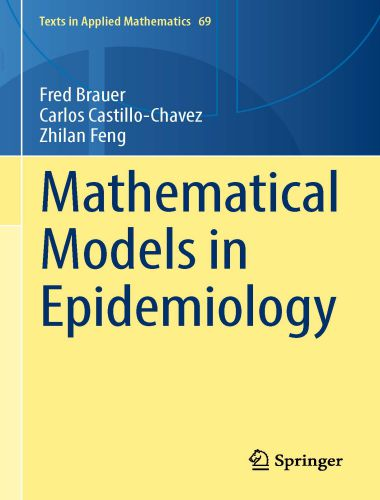# Mathematical Models in Epidemiology

by Fred Brauer, Carlos Castillo-Chavez, Zhilan Feng
October 2019The book is a comprehensive, self-contained introduction to the mathematical modeling and analysis of disease transmission models. It includes (i) an introduction to the main concepts of compartmental models including models with heterogeneous mixing of individuals and models for vector-transmitted diseases, (ii) a detailed analysis of models for important specific diseases, including tuberculosis, HIV/AIDS, influenza, Ebola virus disease, malaria, dengue fever and the Zika virus, (iii) an introduction to more advanced mathematical topics, including age structure, spatial structure, and mobility, and (iv) some challenges and opportunities for the future.

There are exercises of varying degrees of difficulty, and projects leading to new research directions. For the benefit of public health professionals whose contact with mathematics may not be recent, there is an appendix covering the necessary mathematical background. There are indications which sections require a strong mathematical background so that the book can be useful for both mathematical modelers and public health professionals.

• A modern description of many important areas of mathematical epidemiology
• Provides an introduction to the formation and analysis of disease transmission models
• Exercise sets and some projects included

The goal of this book is to interest students of mathematics and public health professionals in the modeling of infectious diseases transmission. We believe that some knowledge of disease transmission models can give useful insights to epidemiologists and that there are interesting mathematical problems in such models. The mathematical background necessary for studying this book is a knowledge of calculus, some matrix theory, and some ordinary differential equations, speciﬁcally approximate and qualitative methods.

Our emphasis is on describing the mathematical results being used and showing how to apply them rather than on detailed proofs. We hope that eventually the education of biologists and public health professionals will include these mathematical topics in the ﬁrst 2 years of university so that this book can be accessible to upper-level undergraduate students.

[expand title=” “]

Get Mathematical Models in Epidemiology

[/expand]